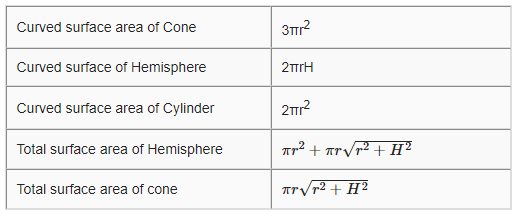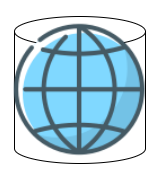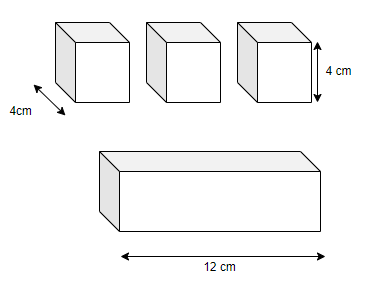# Surface area and volume class 9 extra questions

Given below are the Class 9 MathsExtra questions and Important Questions for Surface area and volume
(a) Concepts questions
(b) Calculation problems
(c) Multiple choice questions
(e) Fill in the blank's

## Fill in the blank

Question 1
(a) A cylinder radius is doubled and height is halved, the curved surface area of the cylinder will ____ (Increase/decrease/remain same)
(b) The radius of the sphere is 7 cm, the surface area of the sphere is____ (616 cm2 / 700 cm2/ 800 cm2)
(c) Three cubes whose sides are 6 cm, 8 cm and 10 cm. They are melted and form a big cube. The volume of the big cube is ___ (1800cm3/1728 cm3)
(d) The total surface area of a hemisphere of radius 10 cm using value of π=3.14 is _____(956 cm2/942 cm2)
(e) A right circular cylinder just encloses a sphere of radius. The ratio of there surface area is ______ (1:1 / 1:2)
(f) The lateral surface area of a cube is 256 m2, the volume is ____ (456 m3/512 m3)
Solution
(a) Remain same
CSA= 2πrh
So if r is doubled, h is halved,CSA will remain same
(b) 616 cm2,  Surface area=4πr2
(c) 1728 cm3, V=V1 + V2 + V3=63+ 83 +103
(d) 956 cm2, Total surface area =3πr2
(e) 1:1 In this height of cylinder will be 2r and radius of cylinder will r. So it is equal
(f) 512 m3, Lateral surface area=4a2 and Volume=a3c

## True or False statement

Question 2
(a) A cylinder, hemisphere and cone stand on equal base and same height, the Volume ratio is 3:2:1
(b) The radius of a solid sphere is 24 cm. 8 spheres can be made from it of 12cm radius
(c) radius of the cone is doubled and height is halved, the volume will be halved
(d) A river 10m deep and 40m wide is flowing at the rate of 2m per min. 48000m3 water will flow into the sea from river
(e) A cylinder radius is halved and height is doubled, the volume will become halved
(f) The side of the cube is 4 cm, the diagonal length is  cm
Solution
(a)True.. All of them will have height as r as hemisphere height can be r only. For cylinder  =πr3
Hemisphere=(2/3) πr3
Cone=(1/3) πr3
So ratio is 3:2:1

(b) True. Sphere volume =(4/3) πr3

(c) False. Volume will be doubled. Volume is given by =(1/3)πr2h

(d) True. It is equal to the volume of the cuboid  10m,40m,and 120 m

(e) True.

(f)False

## Multiple choice Questions

Question 3
The length, breadth and height of a room are 12 m, 10 m, and 9m respectively. Find the area of  our walls of room?
(a) 636 m2
(b) 516 m2
(c) 800 m2
(d) 456m2
Solution
Area of the walls is given by =2(BH+LH) +LB=2(90+108)+120=516 m2

Question 4
The plastic paint in a Asian paint container is sufficient to paint an area equal to 93.75m2 How many blocks of dimensions 22.5 cm × 10 cm × 7.5 cm can be painted out of this container
(a) 100
(b) 800
(c) 940
(d) 1000
Solution
Total surface area of one block = 2(lb + bh + lh)
= [2(22.5 ×10 + 10 × 7.5 + 22.5 × 7.5)] cm
= 2(225 + 75 + 168.75) cm2
= (2 × 468.75) cm2
= 937.5 cm2
Let n blocks can be painted out by the paint of the container.
Area of n bricks = (n ×937.5) cm2 = 937.5n cm2
Area that can be painted by the paint of the container = 93.75 m2= 937500 cm2
937500 = 937.5n
n = 1000
Therefore, 1000 blocks can be painted out by the paint of the container

Question 5
Curved surface area of a cone is 308 cm2 and its slant height is 14 cm.
(a) Radius of the cone is 7 cm
(b) total surface area is 462 cm2
(c) Height of the cone is $\sqrt {147}$ cm
(d) None of the above
Solution
Curved surface area=πrl
So r=7 cm
Now total surface area=πr2+πrl=462 cm2

Question 6
Sita had to make a model of cylindrical kaleidoscope for her science project. She wanted to use black chart paper to make the curved surface of the kaleidoscope. What would be the area of chart paper required by her, if she wanted to make a kaleidoscope of length 30cm with a 2.7 cm radius? (Use p=22/7)
(a)1320 cm2
(b) 1400 cm2
(c) 986 cm2
(d) None of these
Solution
Curved surface of cylinder=2πrh

Question 7
The radii of two cones are in the ratio of 2:3 and their heights are in the ratio of 7:3. The ratio of their volumes is
(a) 20:27
(b) 28:27
(c) 28:27
(d) None of these
Solution
Volume =(1/3)πr2h

Question 8
Find the maximum length of the rod that can be kept in cuboidal box of sides 30cm, 20cm and 10cm.
(a)$\sqrt {1400}$ cm
(b)$2 \sqrt {400}$ cm
(c)$2 \sqrt {300}$ cm
(d) None of these
Solution
Diagonal is the longest length in the cuboid so
$D=\sqrt {L^2+B^2+H^2}$
=$\sqrt {1400}$ cm

Question 9
A box is made entirely of glass panes (including base) held together with tape. It is 3 cm long, 2.5 cm wide and 2.5 cm high. How much of tape is needed for all the 12 edges?
(a) 30cm
(b) 32cm
(c) 40 cm
(d) None of these
Solution
Length of tape=4( L+B+H)=32 cm

Question 10
The curved surface area of a right circular cylinder of height 14 cm is 88 cm2. Find the Volume of the cylinder. Assume π=22/7
(a) 144 cm3
(b) 180 cm3
(c) 176 cm3
(d) None of the above
Solution

## Match the column

Question 11## Subjective Type questions

Question 12
Ramesh has build a cuboidal water tank with lid for his house with each outer edge 1.5m long. He gets the outer surface of tank excluding the base, covered with square tiles of side 25cm. Find how much he would spend for tiles, if cost of the tile is Rs 400 per dozen.
Solution
Surface Area of the cuboidal water tank where tiles to be placed= 5L2= 11.25 m2
Area of 1 Tile= 252=625 cm2=.0625 m2
No of Tiles required=11.25/.0625=180=15 dozen
Cost of tiles will be = 400 * 15=Rs 6000

Question 13
The paint in a certain container is sufficient to point an area equal to 9.375m2. How many bricks of dimensions 22.5cm x 10cm x 7.5cm can be painted out of this container.
Solution
Surface are of 1 Brick= 2(LB+ BH+ LH)=2(225 + 75 + 168.75)=937.5 cm2
Total Brick which can be painted= $\frac {9.375 \times 10^4}{937.5}= 100 Question 14 Bikanerwala sweets shop was planning an order for making cardboard boxes for packing their sweets. Two sizes of boxes were required. The bigger of dimensions 25cm x 20cm x 5cm and smaller of dimensions 15cm x 12cm x 5cm. For all overlaps, 5% of total surface area is required extra. If cost of cardboard is Rs 4 for 1000cm2, find the cost of cardboard required for supplying 250 boxes of each kind. Question 15 The diameter of a roller are 84cm and its length is 120cm. It takes 500 complete revolutions to move once more over to level a playground. Find area of playground in m2? Solution Diameter =84 cm, Therefore radius=42 cm Curved Surface area of cylinder=$2 \pi r h= 2 \times \frac {22}{7} \times 42 \times 120 $=31680 cm2= 3.1680 m2 Area of playground=500 × 3.1680=1584 m2 Question 16 In a hot water heating system, there is a cylindrical pipe of length 28m and diameter 5cm. Find the total radiating surface in system? Solution Diameter =5 cm, Therefore radius=2.5 cm Curved Surface area of cylinder is the radiating surface=$2 \pi r h= 2 \times \frac {22}{7} \times 2.5 \times 2800 =44000$cm2 Question 17 Find (a)the lateral or C.S.A of a closed cylindrical petrol storage tank that is 4.2m in diameter and 4.5m in high (b)how much steel was actually used, if 1/12 of steel actually used was wasted in making the tank. Question 18 You see the frame of lamp shade. It is to be covered with decorative cloth. The frame has a base diameter of 20cm and height of 30cm. A margin of 2.5cm is total given for folding it over top and bottom of frame How much cloth is required for covering the lamp shade? Question 19 What length of tarpaulin 3m wide will be required to make a conical tank of height 8m and base radius 6m. Assume that the extra length margins and wastage n cutting is approximately 20cm. (Use = 3.14) Question 20 A Birthday cap is in form of right circular cone of radius 7cm and height 24cm. Find area of sheet required to make 10 such caps? Question 21 A bus stop is barricaded from remaining part of road, by using 50 hollow cones made of recycles cardboard. Each cone has a base diameter of 4cm and height 1m. If outer side of earn cone is to be painted and cost of painting is Rs 12/m2. What will be the cost? ( = 3.14 and take 1.04 = 1.02) Question 22 A hemispherical dome of a building needs to be painted. If circumference of base of dome is 17.6m, find the cost of painting it, given cost of painting is Rs. 5/200 cm2. Question 23 The diameter of moon is approximately one – fourth diameter of earth. Find the ratio of their surface areas. and What fraction of the volume of the earth is the volume of the moon? Solution Let d be diameter of earth Surface area of earth=$ \pi d^2$Diameter of Moon=$\frac {1}{4}d$Surface area of Moon=$\pi D^2= \frac {1}{16} \pi d^2\frac {\text{surface area of Moon}}{\text{surface area of earth}}= \frac {1}{16}$Volume of earth=$\frac {1}{6} \pi d^3$Surface area of Moon=$\frac {1}{6} \pi D^3= \frac {1}{384} \pi d^2\frac {\text{Volume of Moon}}{\text{Volume of earth}}= \frac {1}{384}$Question 24 A right circular cone just enclosed a sphere of radius r. Find – (a)surface area of sphere (b)curved surface area of cylinder (c)ratio of areas obtained in (i) and (ii) Solution(i) Surface area of a sphere where r is the radius of the sphere =$4 \pi r^2$(ii) Height of the cylinder, =diameter of the sphere=2r And Radius of the cylinder =r CSA of cylinder formula =$2 \pi r h=4 \pi r^2$(iii) Ratio between areas =$\frac {4 \pi r^2}{4 \pi r^2}=1:1$Question 25 A wall of length 10m was to be built across an open ground. The height is 4m and thickness is 24cm. If this wall is to be build up with bricks whose dimensions are 24cm x 12cm x 8cm, how many bricks would be required? Question 26 Three cubes of each side 4cm are joining end to end. Find the surface area of resulting cuboid SolutionSurface of the cuboid will be =$ 4 \times 4 \times 12 + 2 \times 4 \times 4=224$cm2 Question 27 A village having a population of 4000 requires 150 litres of water per head per day. It has a tank measuring 20m x 15m x 6m for how many days will the water of this tank last? Question 28 A godown measures 40m x 25m x 10m. Find the maximum no. of wooden crates each measuring 1.5m x 1.25m x 0.5m that can be stored in godown? Question 29 A river 3m deep and 40m wide is flowing at the rate of 2km/hr. How much water will fall into the sea in a minute? Solution Speed of water in a river=2 km/hr Length of flow of water in 1 minute=$\frac {2}{60} km =\frac {100}{3}m $Therefore Volume of water$=LBH= \frac {100}{3} \times 3 \times 40=4000 m^3 $Volume in litres= 4000 * 1000= 4 × 106 Question 30 The pillars of a temple are cylindrically shaped. If each pillar has a circular base of radius 20cm and height 10m. How much concrete mixture would be required to build 14 such pillars? Solution Volume of single pillar=$\pi r^2 h=.2 \pi$Volume of 14 Pillars=$ 14 \times .2 \pi=8.8 m^3\$

## Summary

This Surface area and volume class 9 extra questions with answers is prepared keeping in mind the latest syllabus of CBSE . This has been designed in a way to improve the academic performance of the students. If you find mistakes , please do provide the feedback on the mail.